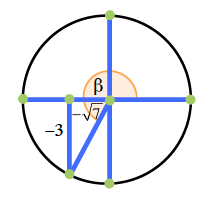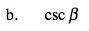### Home > PC > Chapter 4 > Lesson 4.2.2 > Problem4-87

4-87.
1. Given cot β =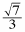and β is in Quadrant III (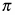< β <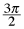), find the following values. Try drawing a triangle where cot β =and find the missing side. Next determine if the value you are finding is positive or negative based on the location of the angle. Homework Help ✎

1. cos β

2. csc β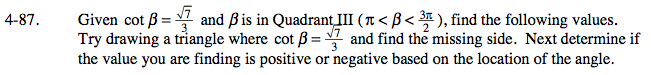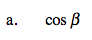What must the signs be for
$\text{cot}\beta=\frac{\text{cos}\beta}{\text{sin}\beta}=\frac{-\sqrt{7}}{-3}$